# Three students

Three students independently try to solve the problem. The first student will solve a similar problem with a probability of 0.6, the second student will solve at a probability of 0.55, and the third will solve at a probability of 0.04. The problem is resolved; what is the probability that the first student has solved it?

p =  0.4963

### Step-by-step explanation: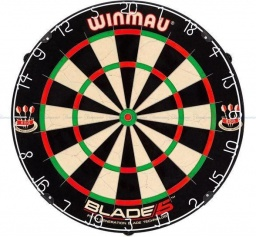Did you find an error or inaccuracy? Feel free to write us. Thank you!Tips to related online calculators
Would you like to compute count of combinations?

## Related math problems and questions:

• Probability of intersectionThree students have a probability of 0.7,0.5 and 0.4 to graduated from university respectively. What is the probability that at least one of them will be graduated?
• The universityAt a certain university, 25% of students are in the business faculty. Of the students in the business faculty, 66% are males. However, only 52% of all students at the university are male. a. What is the probability that a student selected at random in the
• Three subjects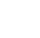In a class of 40 students, 18 passed mathematics, 19 passed accounts, 16 passed economics, 5 mathematics and accounts only, 6 mathematics only, 9 accounts only, 2 accounts and economics only, if each student offered at least one of the subjects. a) how ma
• The accompanyingThe accompanying table gives the probability distribution of the number of courses randomly selected student has registered Number of courses 1 2 3 4 5 6 7 Probability 0.02 0.03 0.1 0.3 0.4 - 0.01 respectively. a) Find the probability of a student registe
• Holidays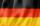Of the 35 students of the class were 7 on holiday in Germany and just as much in Italy. 5 students visited Austria. In none of these countries was 21 students, all three visited by one student. In Italy and Austria were 2 students and in Austria and Germa
• StudentsStudents solve word problems. They received 3 points for each correctly solved task. Four points were deducted for a task that was not solved or incorrectly solved. Tomas solved a total of 15 tasks and scored 24 points. How many tasks did he solve correct
• TestThe teacher prepared a test with ten questions. The student can choose one correct answer from the four (A, B, C, D). The student did not get a written exam at all. What is the probability that: a) He answers half correctly. b) He answers all correctly. c
• travel agency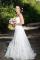Small travel agency offers 5 different tours at honeymoon. What is the probability that the bride and groom choose the same tour (they choose independently)?
• Double probabilityThe probability of success of the planned action is 60%. What is the probability that we will achieve success at least once if this action is repeated twice?
• Fall sum or sameFind the probability that if you roll two dice, it will fall the sum of 10, or the same number will fall on both dice.
• Hazard game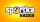In the Sportka hazard game, 6 numbers out of 49 are drawn. What is the probability that we will win: a) second prize (we guess 5 numbers correctly) b) the third prize (we guess 4 numbers correctly)?
• Dices throwsWhat is the probability that the two throws of the dice: a) Six falls even once b) Six will fall at least once
• Utopia IslandThe probability of disease A on the island of Utopia is 40%. The probability of occurrence among the men of this island, which make up 60% of all the population (the rest are women), is 50%. What is the probability of occurrence of A disease among women o
• Internet anywhere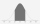In school, 60% of pupils have access to the internet at home. A group of 8 students is chosen at random. Find the probability that a) exactly 5 have access to the internet. b) At least 6 students have access to the internet
• TestingStudents of high school have 10 points for each good solved task. The wrong answer is deducted by 5 points. After solving 20 tasks, student Michael had 80 points. How many tasks did he solve correctly and how many wrong?
• Left handedIt is known that 25% of the population is left-handed. What is the probability that there is a maximum of three left-handers at a seminar where there are 30 participants?
• SalesFrom statistics of sales goods, item A buy 51% of people and item B buys 59% of people. What is the probability that from 10 people buy 2 item A and 8 item B?